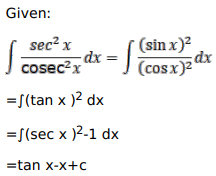# Mark against the correct answer in each of the following:

Question:

Mark $(\sqrt{)}$ against the correct answer in each of the following:

$\int \frac{\sec ^{2} x}{\operatorname{cosec}^{2} x} d x=?$

A. $\tan x+x+C$

B. $\tan x-x+C$

C. $-\tan x+x+C$

D. $-\tan x-x+C$

Solution: